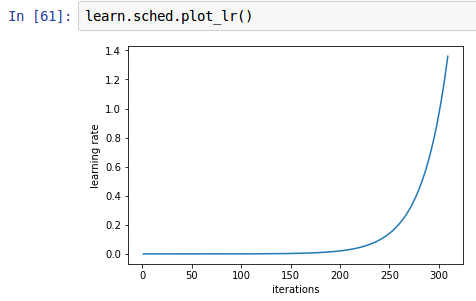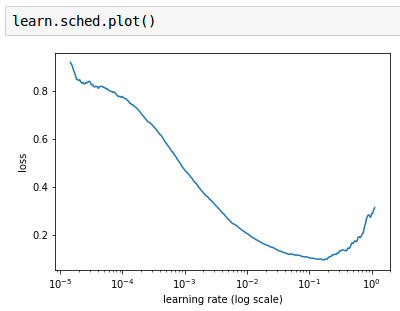# Learn.sched.plot_lr() - why LR increases with respect to the number of iterations?

Hello,

I have difficulties in understanding how `lr.find()` works and what `lr.sched.plot_lr()` is useful for and why the LR is decreasing with respect to the iterations number.

We have almost 23.000 training images. The batch size is 64. So we have 23000/64 ~ 360 batches.
In order to train/find the best LR, we run the training process for each single batch.

When we call `lrf=learn.lr_find()` we have this type of answer

`A Jupyter Widget`

82%|████████▏ | 295/360 [00:01<00:00, 153.04it/s, loss=0.383]

The widget is telling that after 294 batches he found the minimum loss 0.383.

Calling this function `learn.sched.plot_lr()` we can actually see how the LR performs with respect to the iterations. And `x` goes only until 300, because the `lr_find()` stopped at 295.

Q1/ So, why `LR(iteration=100) << LR(iteration 295)`The purpose of this function `learn.sched.plot()` is very clear. We have tried different LRs, for each of them we computed the loss, and we plot the result in order to choose the LR where the loss is decreasing.Q2/ Why we can’t use the `lr_find()` for an already trained model learn.fit(0.01,1)?
We have a trained model, with the computed weights - which has an accuracy of 98%, and a training loss of 0.03 and a validation loss of 0.02.

Given this model after the first iteration it stops. Why it doesn’t try to find a better LR in order to obtain an even smaller loss?

``````learn.lr_find()
``````

Epoch
0% 0/1 [00:00<?, ?it/s]

0%| | 1/360 [00:00<01:27, 4.09it/s, loss=0.0318]

2 Likes

Ah - the key issue I think is a (very understandable!) misunderstanding here. 0.383 is not the minimum loss. It’s the final loss after completing the `lr_find` process, which is much worse that the minimum loss. Based on the `sched.plot()` chart you showed, it appears the minimum loss was around 0.1. This happened at LR around 1e-1 (which is 0.1). Although it’s looking quite flat here, so I’d pick 1e-2 for my LR, since it’s definitely decreasing nicely there.

The learning rate increases by a constant ratio every batch. That’s how the LR finder works - keep increasing the learning rate, and see when it stops improving.

You can - but if the model is already about as good as it can be, it’ll just show that there’s no LR that improves the model! In this case, we’ll need to `unfreeze()` more layers (which we’ll discuss on Monday).

Does that clear things up a bit?

6 Likes

Thanks a lot, now it’s crystal clear!